Pandas Dataframes: Apply Examples

# Pandas Dataframes: Apply Examples

Last updated:

WIP Alert This is a work in progress. Current information is correct but more content may be added in the future.

Pandas version 1.0+ used.

All code available online on this jupyter notebook

## Apply example

To apply a function to a dataframe column, do df['my_col'].apply(function), where the function takes one element and return another value.

import pandas as pd

df = pd.DataFrame({
'name': ['alice','bob','charlie','david'],
'age': [25,26,27,22],
})[['name', 'age']]

# each element of the age column is a string
# so you can call .upper() on it
df['name_uppercase'] = df['name'].apply(lambda element: element.upper())BEFORE: Original dataframe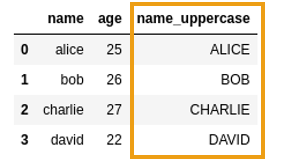AFTER: Created new column using Series.apply()

## Apply example, custom function

To apply a custom function to a column, you just need to define a function that takes one element and returns a new value:

import pandas as pd

df = pd.DataFrame({
'name': ['alice','bob','charlie','david'],
'age': [25,26,27,22],
})

# function that takes one value, returns one value
def first_letter(input_str):
return input_str[:1]

# pass just the function name to apply
df['first_letter'] = df['name'].apply(first_letter)Source dataframe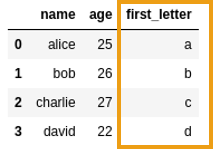Crated a new column passing a
custom function to apply

## Take multiple columns as parameters

Double square brackets return another dataframe instead of a series

To apply a single function using multiple columns, select columns using double square brackets ([[]]) and use axis=1:

import pandas as pd

df = pd.DataFrame({
'name': ['alice','bob','charlie','david'],
'age': [25,26,27,22],
})

# define a function that takes two values, returns 1 value
def concatenate(value_1, value_2):
return str(value_1)+ "--" + str(value_2)

# note the use of DOUBLE SQUARE BRACKETS!
df['concatenated'] = df[['name','age']].apply(
lambda row: concatenate(row['name'], row['age']) , axis=1)Original dataframe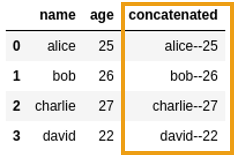Created a new column by applying a function that
takes two columns and concatenates them as strings

## Apply function to row

To apply a dunction to a full row instead of a column, use axis=1 and call apply on the dataframe itself:

Example: Sum all values in each row:

import pandas as pd

df = pd.DataFrame({
'value1': [1,2,3,4,5],
'value2': [5,4,3,2,1],
'value3': [10,20,30,40,50],
'value4': [99,99,99,99,np.nan],
})

def sum_all(row):
return np.sum(row)

# note that apply was called on the dataframe itself, not on columns
df['sum_all'] = df.apply(lambda row: sum_all(row) , axis=1)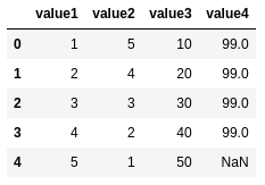Source dataframe where each row contains
observations for one sample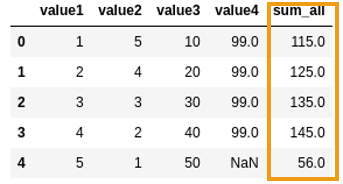Generated a new column by summing all
values in the row, with numpy.sum

## Apply function to column

Just use apply. Example here

## Return multiple columns

To apply a function to a column and return multiple values so that you can create multiple columns, return a pd.Series with the values instead:

Example: produce two values from a function and assign to two columns

import pandas as pd

df = pd.DataFrame({
'name': ['alice','bob','charlie','david','edward'],
'age': [25,26,27,22,np.nan],
})

def times_two_times_three(value):
value_times_2 = value*2
value_times_3 = value*3

return pd.Series([value_times_2,value_times_3])

# note that apply was called on age column
df[['times_2','times_3']]= df['age'].apply(times_two_times_three)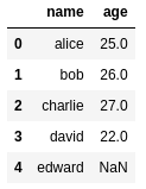Source dataframe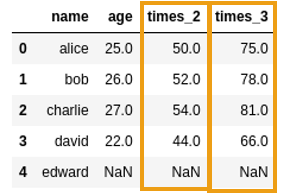Modified dataframe with two new columns,
both returned by apply

## Apply function in parallel

If you have costly operations you need to perform on a dataframe, (e.g. text preprocessing), you can split the operation into multiple cores to decrease the running time:

import multiprocessing

import numpy as np
import pandas as pd

# how many cores do you have?
NUM_CORES=8

# split the dataframe into chunks, depending on hoe many cores you have
df_chunks = np.array_split(df ,NUM_CORES)

# this is a function that takes one dataframe chunk and returns
# the processed chunk (for example, adding processed columns)
def process_df(input_df):
# copy the dataframe to prevent mutation in place
output_df = input_df.copy()

# apply a function to every row *in this chunk*
output_df['new_column'] = output_df.apply(some_function, axis=1)

return output_df

with multiprocessing.Pool(NUM_CORES) as pool:
# process each chunk in a separate core and merge the results
full_output_df = pd.concat(pool.map(process_df, df_chunks), ignore_index=True)


## Vectorization and Performance

TODO


## map vs apply

map() apply()
Series functionSeries function
and Dataframe function
Returns new SeriesReturns new dataframe,
possibly with a single column
Can only be applied to a single
column (one element at
a time)
Can be applied to multiple
columns at the same time
Operates on array elements,
one at a time
Operates on whole columns or rows
Very slow, no better than a
Python for loop
Much faster when you can use
numpy vectorized functions